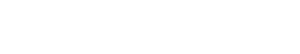# Corpus topic probabilities

Corpus topic probabilities refers to the probability that a given topic will be present in a document, based on the presence of specific keywords in that document. This metric is often used to compare the similarity of two documents, or to determine which topics are most important in a given corpus of documents.

Topic modeling is a statistical technique for finding the abstract “topics” that occur in a collection of documents. Latent Dirichlet allocation (LDA) is a popular algorithm for topic modeling, and it can be used to estimate corpus topic probabilities. LDA represents each document as a mixture of topics, and each topic is represented as a distribution over words. The mixing proportions for each document (i.e., thetopic probabilities) can be used to compare the similarity of documents or to determine which topics are most important in a corpus.

There are other ways to calculate topic probabilities, but LDA is a popular method due to its flexibility and ease of use. There are many software packages available that can perform LDA, including R, Python, and MATLAB.

When calculating topic probabilities, it is important to keep in mind that the results will be sensitive to the choice of priors (i.e., the set of parameters that defines the probability distribution over topics). Therefore, it is advisable to use multiple runs of LDA with different priors in order to get a robust estimate of topic probabilities.

In conclusion, corpus topic probabilities is a statistical measure that can be used to compare the similarity of documents or to determine which topics are most important in a corpus. LDA is a popular algorithm for calculating topic probabilities, but it is important to use multiple runs of LDA with different priors in order to get a robust estimate.

## How to compute Corpus topic probabilities

P(topic|document) = P(topic) * \prod_{i=1}^{N}P(w_i|topic)

where:

P(topic|document) is the probability that a topic is present in a document

P(topic) is the prior probability of the topic

P(w_i|topic) is the likelihood of word w_i given the topic

N is the number of words in the document.Unlock the power of actionable insights with AI-based natural language processing.